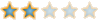# [分享]文字外框圓角(VBA)##[分享]文字外框圓角(VBA)`Function AddTextBox(ByVal txtobj As Object)Dim ldpt(2) As DoubleDim rupt(2) As DoubleSet entobj = txtobjCall entobj.GetBoundingBox(Min, Max)r = Sqr((Max(0) - Max(0)) ^ 2 + (Max(1) - Min(1)) ^ 2)ldpt(0) = Min(0) - 0.2 * r: ldpt(1) = Min(1) - 0.2 * rrupt(0) = Max(0) + 0.2 * r: rupt(1) = Max(1) + 0.2 * r Set AddTextBox = PlotRecFillet(ldpt, rupt, 0.4 * r)End FunctionFunction PlotRecFillet(ByRef LeftLowerPoint() As Double, ByRef RightUpperPoint() As Double, ByVal r As Double)Dim vertices(9 * 3 - 1) As DoubleDim Rec As ObjectX1 = LeftLowerPoint(0): Y1 = LeftLowerPoint(1)X2 = RightUpperPoint(0): Y2 = RightUpperPoint(1)vertices(0) = X1: vertices(1) = Y1 + rvertices(3) = X1 + r: vertices(4) = Y1vertices(6) = X2 - r: vertices(7) = Y1vertices(9) = X2: vertices(10) = Y1 + rvertices(12) = X2: vertices(13) = Y2 - rvertices(15) = X2 - r: vertices(16) = Y2vertices(18) = X1 + r: vertices(19) = Y2vertices(21) = X1: vertices(22) = Y2 - rvertices(24) = X1: vertices(25) = Y1 + rSet tmp = thisdrawing.modelspace.AddPolyLine(vertices)tmp.SetBulge 0, 0.4tmp.SetBulge 2, 0.4tmp.SetBulge 4, 0.4tmp.SetBulge 6, 0.4Set PlotRecFillet = tmpEnd Function`##回復： [分享]文字外框圓角(VBA)

`Sub Example_AddTextBox()    Dim ssetObj As AcadSelectionSet    Set ssetObj = ThisDrawing.SelectionSets.Add("SSET")    Dim gpCode(0) As Integer    Dim dataValue(0) As Variant    gpCode(0) = 0    dataValue(0) = "TEXT"        Dim groupCode As Variant, dataCode As Variant    groupCode = gpCode    dataCode = dataValue    ssetObj.SelectOnScreen groupCode, dataCode    For Each i In ssetObj        AddTextBox (i)    Next    ssetObj.DeleteEnd Sub`naruto018##回復： [分享]文字外框圓角(VBA)##回復： [分享]文字外框圓角(VBA)

LISP的凸出度函數

`;;;(Angle->Bulge 起始角度 終止角度);;;回傳：凸度(defun Angle->Bulge ( a1 a2 / bk )    ((lambda (a) (/ (sin a) (cos a)))          (/ (rem (+ pi pi (- a2 a1)) (+ pi pi)) 4.0)        )    );_結束`

_\$ (Angle->Bulge (* PI 1.0) (* PI 1.5))
0.414214naruto018